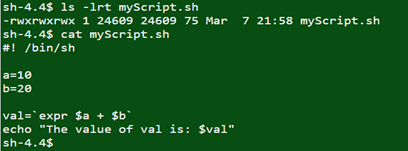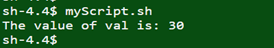# Arithmetic Operators in Unix

#### Prev     Next

Arithmetic Operators in Unix: In Unix we have capability to add, subtract, multiply, division of arithmetic values.

• + (Addition) – To add values on any side of the operator.

Ex:

`expr \$a + \$b`

• -(Subtraction) – To subtract right hand operand from left hand operand.

Ex:

`expr \$a - \$b`

• *(Multiplication) – To multiply the values on either side of the operator.

Ex:

`expr \$a \* \$b`

Note:

* is the special character in Unix and whenever we use special character, we should prefix with the symbol \ .

• / (Division) – To divide the left-hand operand by right hand operand.

Ex:

`expr \$b / \$a`

• % (Modulus) – To divide the left-hand operand by right hand operand and returns remainder

Ex:

`expr \$b % \$a`

• = (Assignment) – To assign the right operand in left operand. The value of b will be assigned to variable a.

Ex:

a = \$b

• == (Equality) – To compare the 2 numbers. If both are same then it will return True else it will return False.

Ex:

[ \$a == \$b ]

• != (Not Equality) – To compare the two numbers. If both are different then it will return true.

Ex:

[ \$a != \$b ]

In Unix, it is very important to know that all the conditional expressions should be inside the square braces with the space.

For Ex:

[ \$a == \$b ] is correct.

[\$a==\$b] is incorrect.

Ex:# Execution Results: